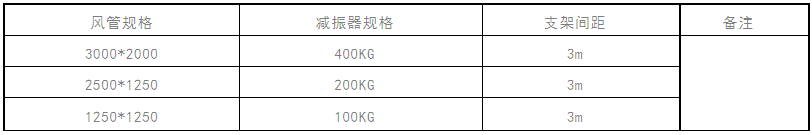首页 >> 公司新闻 >>公司新闻 >> 中央空调管道减振器选型计算书-减振器哪家便宜为你呈现

# 中央空调管道减振器选型计算书-减振器哪家便宜为你呈现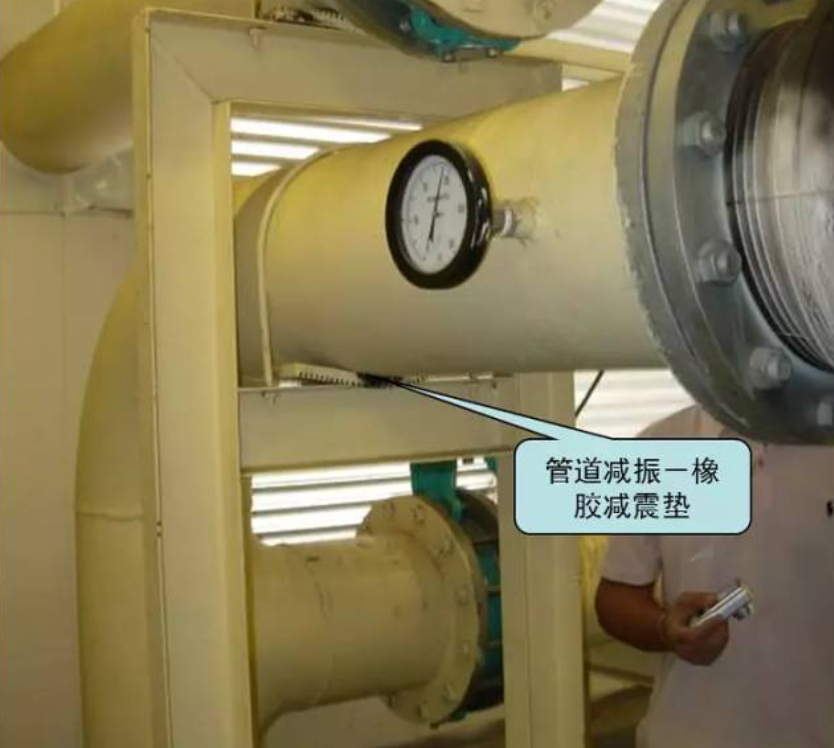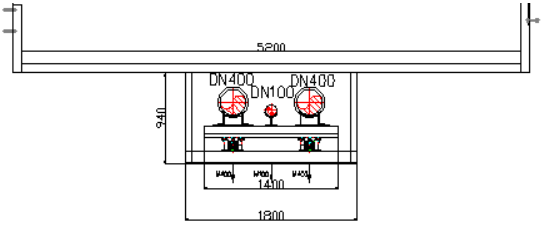DN400的水管含水重量为M400=222Kg；

DN100的水管含水重量为M100=20Kg；

DN400需要的减振器大小为A=（222*6*2+20*6+19）*1.5/2=2103 Kg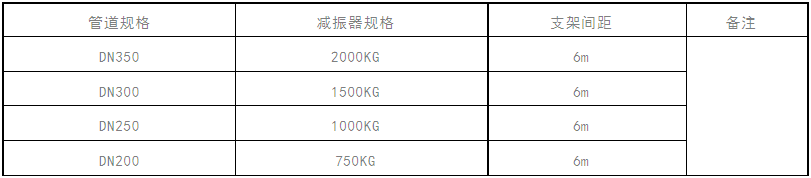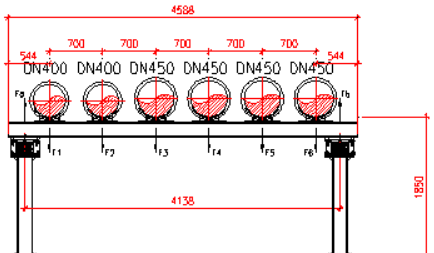DN400的水管含水重量为M400=222Kg；

DN450的水管含水重量为M450=270Kg；

H钢横梁重量为MH =233Kg；平均分至每个受力点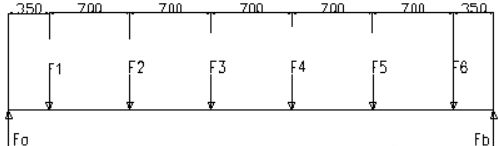F1= F2=222*5.4+39=1238Kg

F3= F4= F5= F6=270*5.4+39=1497Kg

Fb*4.2=F1*0.35+ F2*1.05+ F3*1.75+F4*2.45+ F5*3.15+ F6*3.85

Fb=4405Kg   Fa= F1+ F2+ F3+ F4+ F5+ F6- Fb=4060 Kg

A点减振器型号为7200 Kg，B点减振器型号为7200 Kg。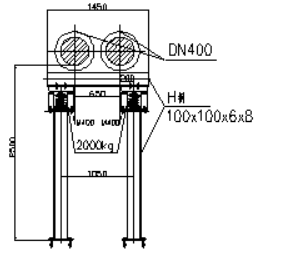DN400的水管含水重量为M400=222Kg；

H钢横梁重量为MH=25Kg

DN400需要的减振器大小为A=（222*5.4*2+25）*1.5/2=1817Kg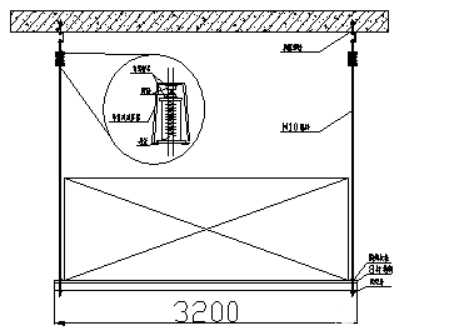3000*1000mm风管采用厚为1.2mm的镀锌钢板，加固框采用4#角钢，4#角钢的每米重量为3.77Kg，法兰采用3#扁钢，每米扁钢的重量为0.707 Kg，支架间距L=3000mm。 每两个支架间距内有3个加固框，3组法兰。

Ma=（3+1）*2*3*1.2*7.85=226.08Kg；

M=（226.08+90.38+16.968+25.744）*1.5/2=269.379 Kg